# Contents

This vignette show how to use MOFA including initialization, training and down-stream analyses. For illustration we use the CLL data which is used in the MOFA publication.

``````library(MultiAssayExperiment)
library(MOFA)

# 1 Step 1: Load data and create MOFA object

There are two options to input data to MOFA:

• Option 1: base R approach using a list of matrices
• Option 2: Bioconductor approach using the MultiAssayExperiment framework

## 1.1 Option 1: base R approach

If using the base R approach, you simply need to provide a list of matrices where features are rows and samples are columns. Importantly, the samples need to be aligned. Missing values/assays should be filled with NAs.

``````data("CLL_data")
MOFAobject <- createMOFAobject(CLL_data)``````
``````## Creating MOFA object from a list of matrices
##  please make sure that samples are stored in the columns and features in the rows...``````
``MOFAobject``
``````## Untrained MOFA model with the following characteristics:
##   Number of views: 4
##   View names: Drugs Methylation mRNA Mutations
##   Number of features per view: 310 4248 5000 69
##   Number of samples: 200``````

## 1.2 Option 2: Bioconductor approach

If using the Bioconductor approach, you need to provide or create a MultiAssayExperiment object and then use it to build the MOFA object. For example, starting from a list of matrices where features are rows and samples are columns, this can be easily constructed as follows:

``````# Load data
# import list with mRNA, Methylation, Drug Response and Mutation data.
data("CLL_data")

# check dimensionalities, samples are columns, features are rows
lapply(CLL_data, dim) ``````
``````## \$Drugs
##  310 200
##
## \$Methylation
##  4248  200
##
## \$mRNA
##  5000  200
##
## \$Mutations
##   69 200``````
``````# Load sample metadata: Sex and Diagnosis
data("CLL_covariates")
``````##      Gender Diagnosis
## H045      m       CLL
## H109      m       CLL
## H024      m       CLL
## H056      m       CLL
## H079      m       CLL
## H164      f       CLL``````
``````# Create MultiAssayExperiment object
mae_CLL <- MultiAssayExperiment(
experiments = CLL_data,
colData = CLL_covariates
)

# Build the MOFA object
MOFAobject <- createMOFAobject(mae_CLL)
MOFAobject``````
``````## Untrained MOFA model with the following characteristics:
##   Number of views: 4
##   View names: Drugs Methylation mRNA Mutations
##   Number of features per view: 310 4248 5000 69
##   Number of samples: 200``````

## 1.3 Overview of training data

The function `plotDataOverview` can be used to obtain an overview of the data stored in the object for training. For each sample it shows in which views data are available. The rows are the different views and columns are samples. Missing values are indicated by a grey bar.

``plotDataOverview(MOFAobject)``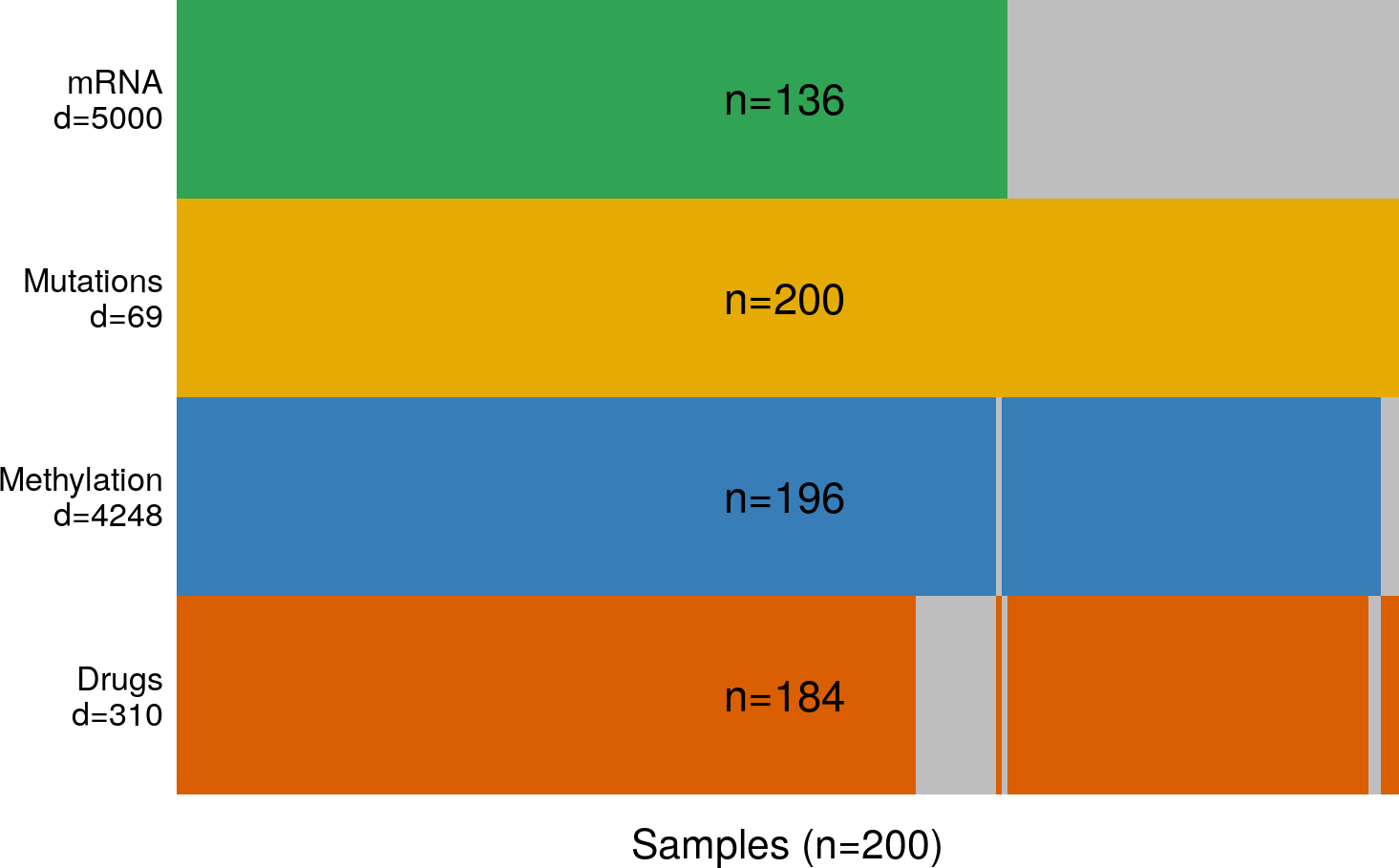# 2 Step 2: Fit the MOFA model

The next step is to fit the model. This part of the pipeline is implemented in Python, so first of all make sure you have the corresponding package installed (see installation instructions and read the FAQ if you have problems).

## 2.1 Define options

### 2.1.1 Define data options

The most important options the user needs to define are:

• scaleViews: logical indicating whether to scale views to have unit variance. As long as the scale of the different data sets is not too high, this is not required. Default is `FALSE`.

• removeIncompleteSamples: logical indicating whether to remove samples that are not profiled in all omics. The model can cope with missing assays, so this option is not required. Default is `FALSE`.

``````DataOptions <- getDefaultDataOptions()
DataOptions ``````
``````## \$scaleViews
##  FALSE
##
## \$removeIncompleteSamples
##  FALSE``````

### 2.1.2 Define model options

Next, we define model options. The most important are:

• numFactors: number of factors (default is 0.5 times the number of samples). By default, the model will only remove a factor if it explains exactly zero variance in the data. You can increase this threshold on minimum variance explained by setting `TrainOptions\$dropFactorThreshold` to a value higher than zero.

• likelihoods: likelihood for each view. Usually we recommend gaussian for continuous data, bernoulli for binary data and poisson for count data. By default, the model tries to guess it from the data.

• sparsity: do you want to use sparsity? This makes the interpretation easier so it is recommended (Default is `TRUE`).

``````ModelOptions <- getDefaultModelOptions(MOFAobject)
ModelOptions\$numFactors <- 25
ModelOptions``````
``````## \$likelihood
##       Drugs Methylation        mRNA   Mutations
##  "gaussian"  "gaussian"  "gaussian" "bernoulli"
##
## \$numFactors
##  25
##
## \$sparsity
##  TRUE``````

### 2.1.3 Define training options

Next, we define training options. The most important are:

• maxiter: maximum number of iterations. Ideally set it large enough and use the convergence criterion `TrainOptions\$tolerance`.

• tolerance: convergence threshold based on change in the evidence lower bound. For an exploratory run you can use a value between 1.0 and 0.1, but for a “final” model we recommend a value of 0.01.

• DropFactorThreshold: hyperparameter to automatically learn the number of factors based on a minimum variance explained criteria. Factors explaining less than `DropFactorThreshold` fraction of variation in all views will be removed. For example, a value of 0.01 means that factors that explain less than 1% of variance in all views will be discarded. By default this it zero, meaning that all factors are kept unless they explain no variance at all.

``````TrainOptions <- getDefaultTrainOptions()

# Automatically drop factors that explain less than 2% of variance in all omics
TrainOptions\$DropFactorThreshold <- 0.02

TrainOptions\$seed <- 2017

TrainOptions``````
``````## \$maxiter
##  5000
##
## \$tolerance
##  0.1
##
## \$DropFactorThreshold
##  0.02
##
## \$verbose
##  FALSE
##
## \$seed
##  2017``````

## 2.2 Prepare MOFA

`prepareMOFA` internally performs a set of sanity checks and fills the `DataOptions`, `TrainOptions` and `ModelOptions` slots of the `MOFAobject`

``````MOFAobject <- prepareMOFA(
MOFAobject,
DataOptions = DataOptions,
ModelOptions = ModelOptions,
TrainOptions = TrainOptions
)``````
``## Checking data options...``
``## Checking training options...``
``## Checking model options...``

Optionally, we can choose to regress out some (technical) covariates before training, using a simple linear model. For example, here we can choose to remove the effect of sex. Ideally, all undesired sources of variation should be removed a priori from the model. The reason ebing that, if strong technical factors exist, the model will “focus” on capturing the variability driven by the technical factors, and small sources of biological variability could be missed.
(Note: uncomment and running the function below will lead to a slight modification of the results)

``````# MOFAobject <- regressCovariates(
#   object = MOFAobject,
#   views = c("Drugs","Methylation","mRNA"),
#   covariates = MOFAobject@InputData\$Gender
# )``````

## 2.3 Run MOFA

Now we are ready to train the `MOFAobject`, which is done with the function `runMOFA`. This step can take some time (around 15 min with default parameters). For illustration we provide an existing trained `MOFAobject`.
IMPORTANT NOTE: The software has evolved since the original publication and the results are not 100% reproducible with the last versions. Yet, the output should be very similar (if not improved) to the pre-existent model.

``MOFAobject <- runMOFA(MOFAobject)``
``````# Loading an existing trained model
filepath <- system.file("extdata", "CLL_model.hdf5", package = "MOFAdata")

``##  "Could not load the feature intercepts. Your features will be centered to zero mean"``
``MOFAobject``
``````## Trained MOFA model with the following characteristics:
##   Number of views: 4
##  View names: Drugs Methylation Mutations mRNA
##   Number of features per view: 310 4248 69 5000
##   Number of samples: 200
##   Number of factors: 10``````

# 3 Step 3: Analyse a trained MOFA model

After training, we can explore the results from MOFA. Here we provide a semi-automated pipeline to disentangle and characterize all the identified sources of variation (the factors).

Part 1: Disentangling the heterogeneity

Calculation of variance explained by each factor in each view. This is probably the most important plot that MOFA generates, as it summarises the entire heterogeneity of the dataset in a single figure. Here we can see in which view a factor explains variation which can guide further characterisation of the factors by investigating the weights in those views.

Part 2: Characterization of individual factors

• Feature set enrichment analysis (where set annotations are present, e.g. gene sets for mRNA views).
• Ordination of samples by factors to reveal clusters and/or gradients: this is similar to what is traditionally done with Principal Component Analysis or t-SNE.

Other analyses, including imputation of missing values and clustering of samples are also available. See below for a short illustration of these functionalities. In addition, the factors can be used in further analyses, for example as predictors, e.g. for predicting clinical outcome or classifying patients, or to control for unwanted sources of variation. Vignettes illustrating this are coming soon.

## 3.1 Part 1: Disentangling the heterogeneity: calculation of variance explained by each factor in each view

This is done by `calculateVarianceExplained` (to get the numerical values) and `plotVarianceExplained` (to get the plot). The resulting figure gives an overview of which factors are active in which view(s). If a factor is active in more than one view, this means that is capturing shared signal (co-variation) between features of different data modalities.
Here, for example Factor 1 is active in all data modalities, while Factor 4 is specific to mRNA.

``````# Calculate the variance explained (R2) per factor in each view
r2 <- calculateVarianceExplained(MOFAobject)
r2\$R2Total``````
``````##       Drugs Methylation   Mutations        mRNA
##   0.4090497   0.2341916   0.2415335   0.3822602``````
``````# Variance explained by each factor in each view
``````##            Drugs  Methylation    Mutations       mRNA
## LF1 0.1507029688 1.732398e-01 1.537989e-01 0.07519230
## LF2 0.0350660758 5.844575e-03 8.195112e-02 0.04699171
## LF3 0.1123216630 3.789900e-05 2.242679e-05 0.01488067
## LF4 0.0001812843 1.925074e-04 1.934564e-07 0.09061719
## LF5 0.0605883335 1.837185e-05 3.587202e-06 0.02860510
## LF6 0.0340251701 1.181410e-05 3.004497e-06 0.04850234``````
``````# Plot it
plotVarianceExplained(MOFAobject)``````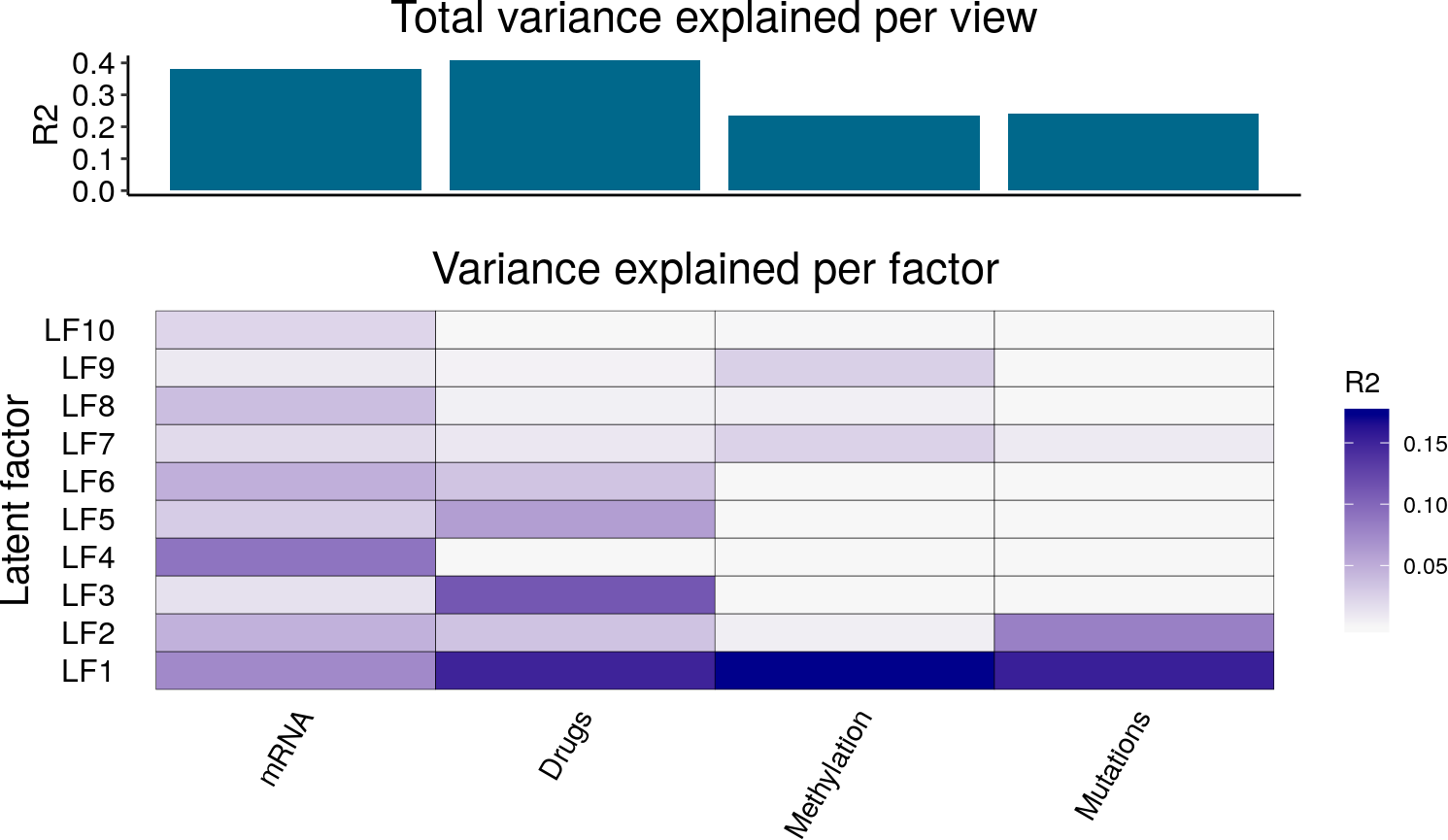## 3.2 Part 2: Characterisation of individual factors

### 3.2.1 Inspection of top weighted features in the active views

To get an overview of the weights across all factors in a given view you can use the `plotWeightsHeatmap` function. For example, here we plot all weights from all factors in the Mutations data:

``````plotWeightsHeatmap(
MOFAobject,
view = "Mutations",
factors = 1:5,
show_colnames = FALSE
)``````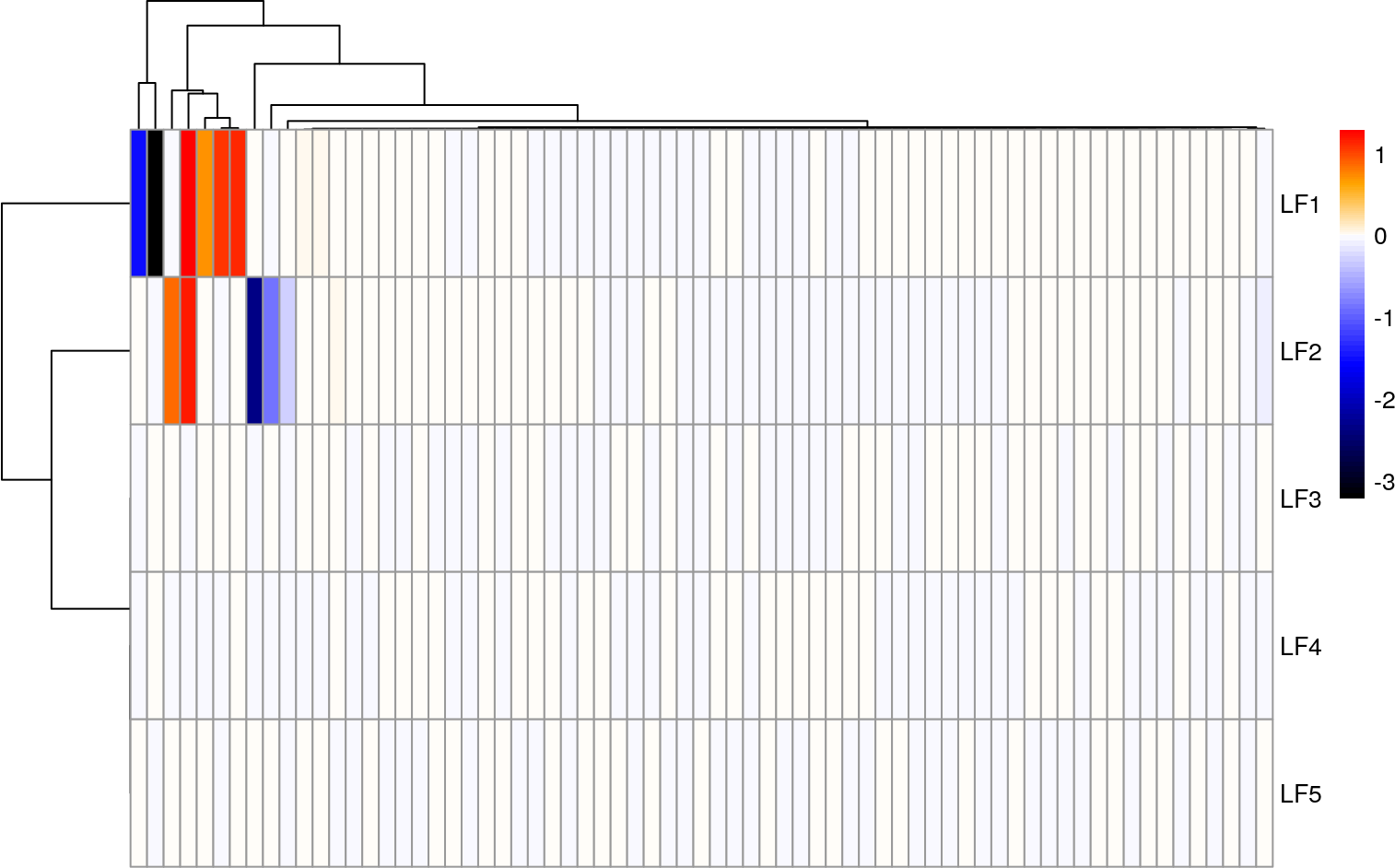We observe that Factors 1 and 2 have large non-zero weights. To explore a given factor in more detail we can plot all weights for a single factor using the `plotWeights` function. For example, here we plot all weights from Factor 1 in the Mutation data. With `nfeatures` we can set how many features should be labelled (`manual` let’s you specify feautres manually to be labelled in the plot.)

``````plotWeights(
MOFAobject,
view = "Mutations",
factor = 1,
nfeatures = 5
)``````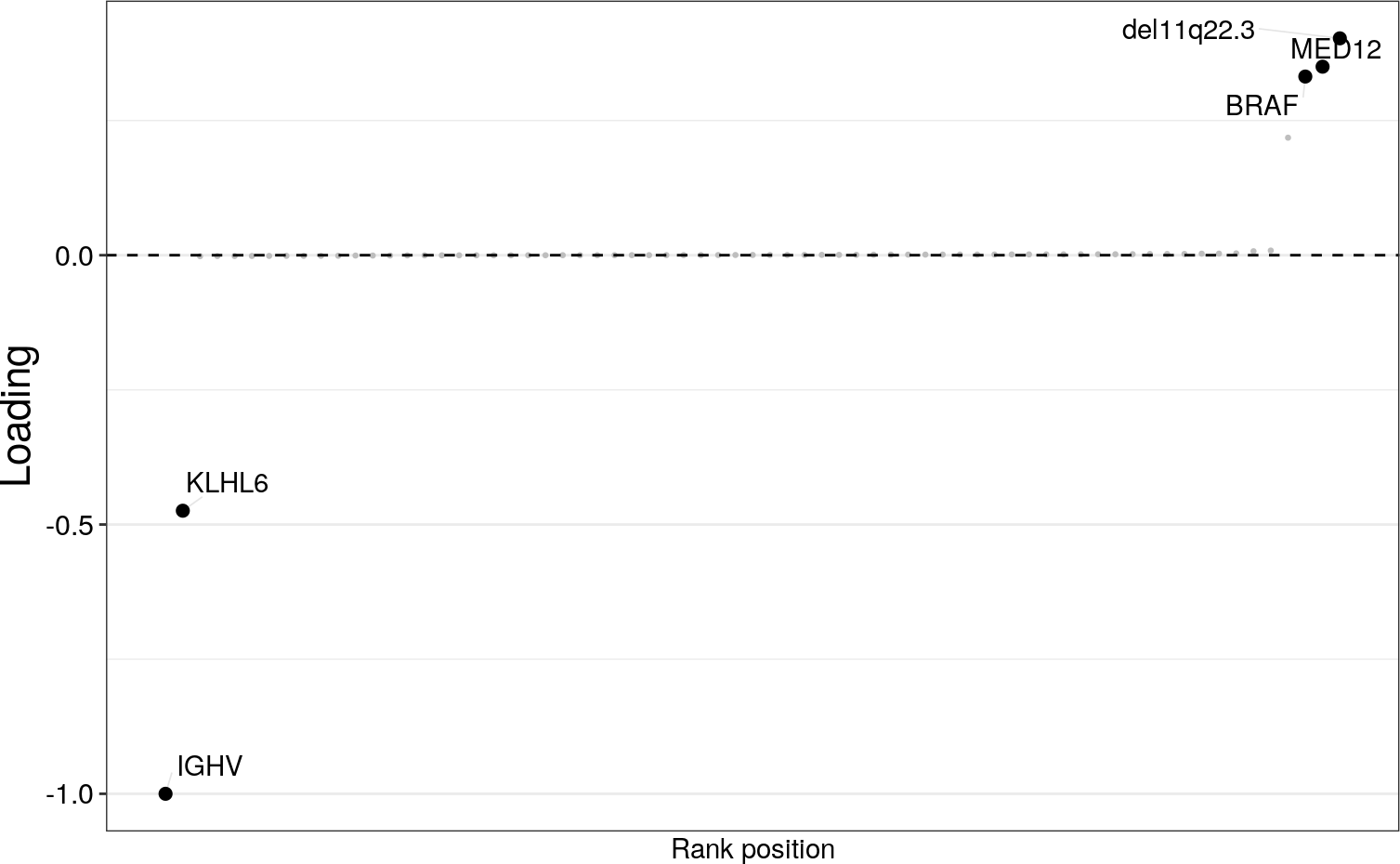``````plotWeights(
MOFAobject,
view = "Mutations",
factor = 1,
nfeatures = 5,
manual = list(c("BRAF"),c("MED12")),
color_manual = c("red","blue")

)``````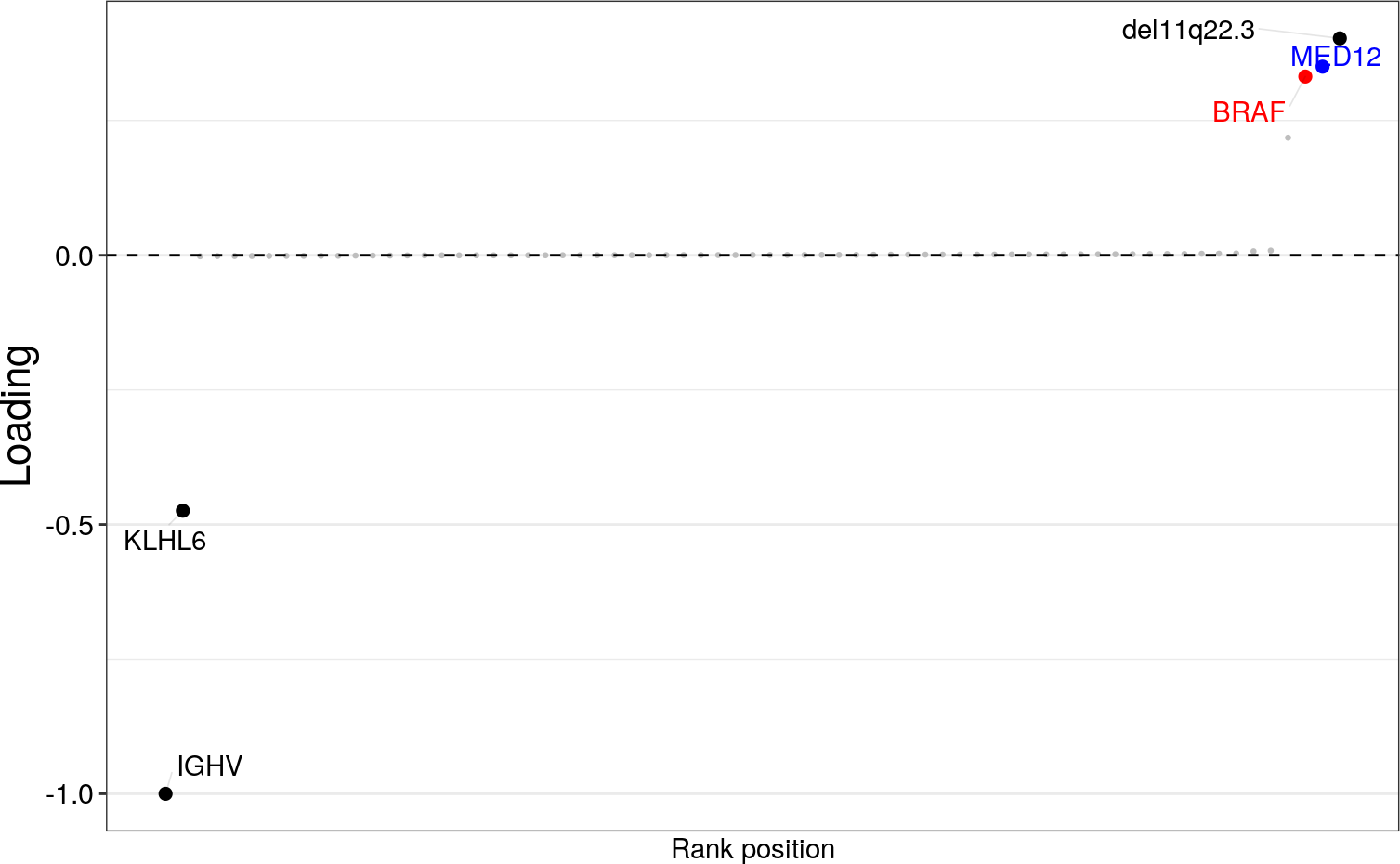Features with large (absolute) weight on a given factor follow the pattern of covariation associated with the factor. In our case, this reveals a strong link to the IGHV status, hence recovering an important clinical marker in CLL (see our paper for details on the biological interpretation.) Note that the sign of the weight can only be interpreted relative to the signs of other weights and the factor values.

If you are only interested in looking at only the top features you can use the `plotTopWeights` function.
For example, here we plot the mutations with largest loadings on Factor 1. The sign on the right indicates the direction of the loading (positive/negative).

``````plotTopWeights(
MOFAobject,
view="Mutations",
factor=1
)``````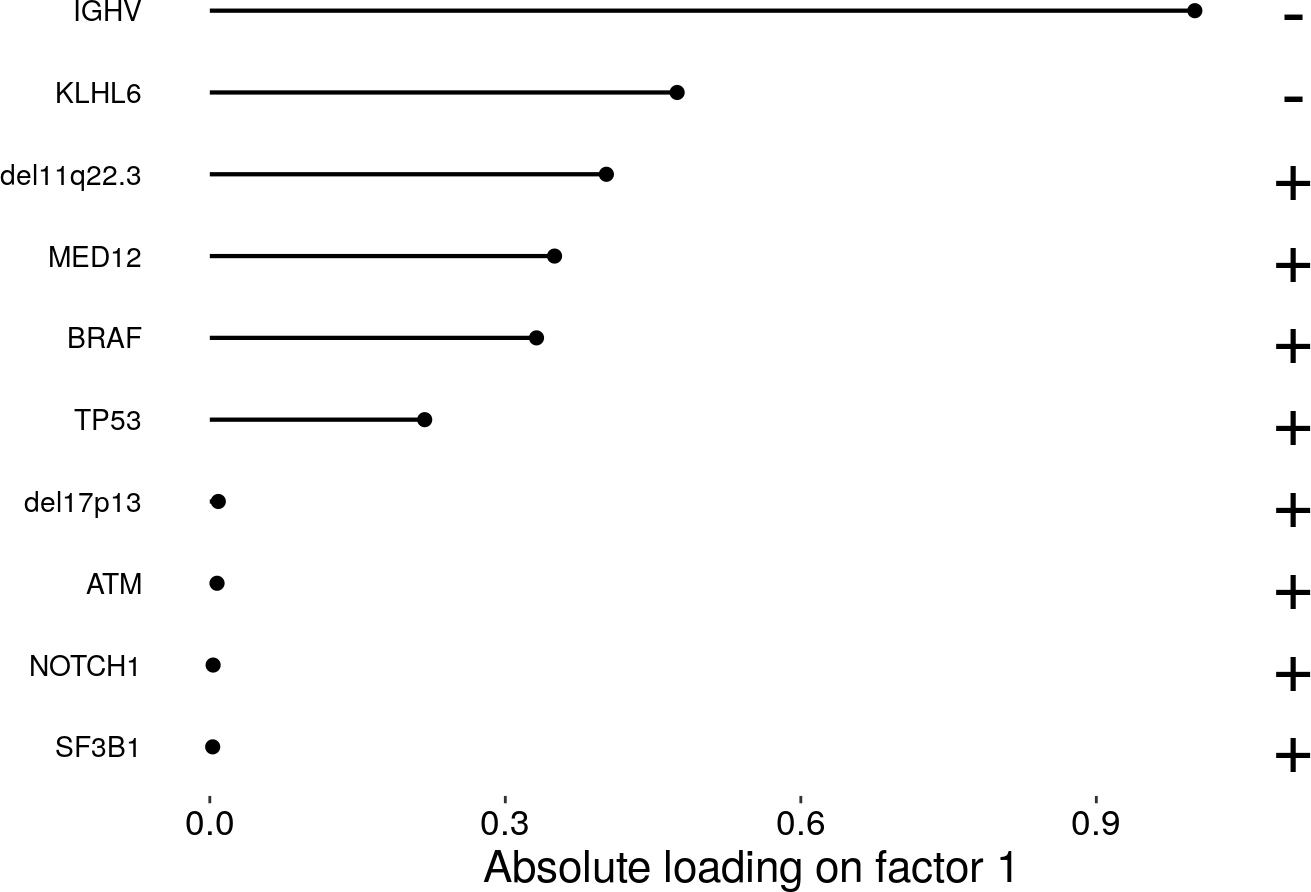Again, features with large weight in a given factor means that they follow the pattern of covariation associated with the factor. For example, here the factor is associated with the B-cell of tumour’s origin, consistent with a large weight on the IGHV status (see our manuscript for more details).

From the previous plots, we can clearly see that Factor 1 is associated to IGHV status. As the variance decomposition above told us that this factor is also relevant on all the other data modalities we can investigate its weights on other modalities, e.g. mRNA, to make connections of the IGHV-linked axes of variation to other molecular layers.

``````plotTopWeights(
MOFAobject,
view = "mRNA",
factor = 1
)``````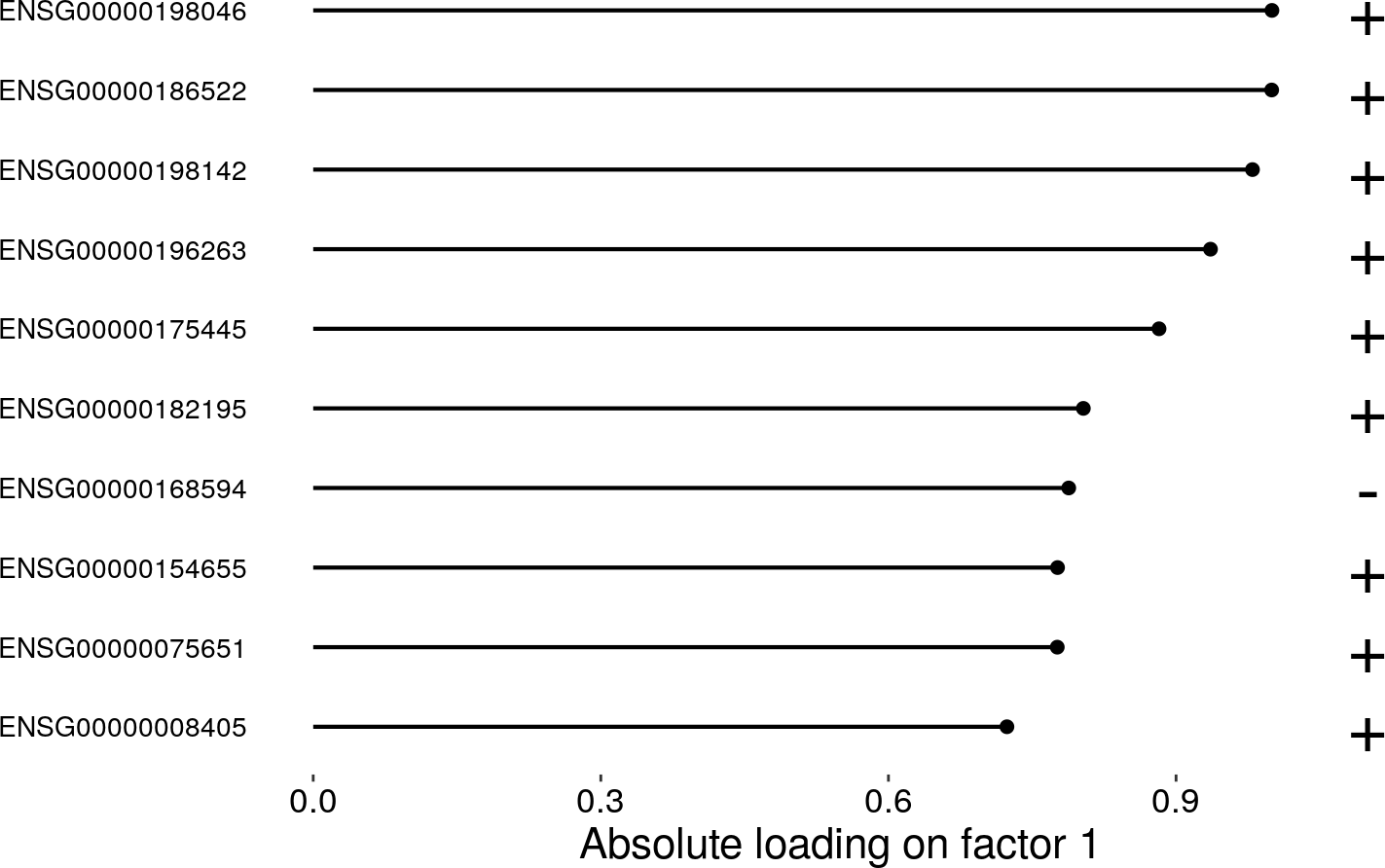Finally, instead of looking at an “abstract” weight, it is useful to observe the coordinated heterogeneity of the top features in the original data. This can be done using the `plotDataHeatmap` function. In this plot samples (in rows) are ordered according to their value on the factor (here Factor 1). Here, this shows clear patterns of the samples’ gene expression in the 20 top weighted genes along the factor.

``````plotDataHeatmap(
MOFAobject,
view = "mRNA",
factor = 1,
features = 20,
show_rownames = FALSE
)``````